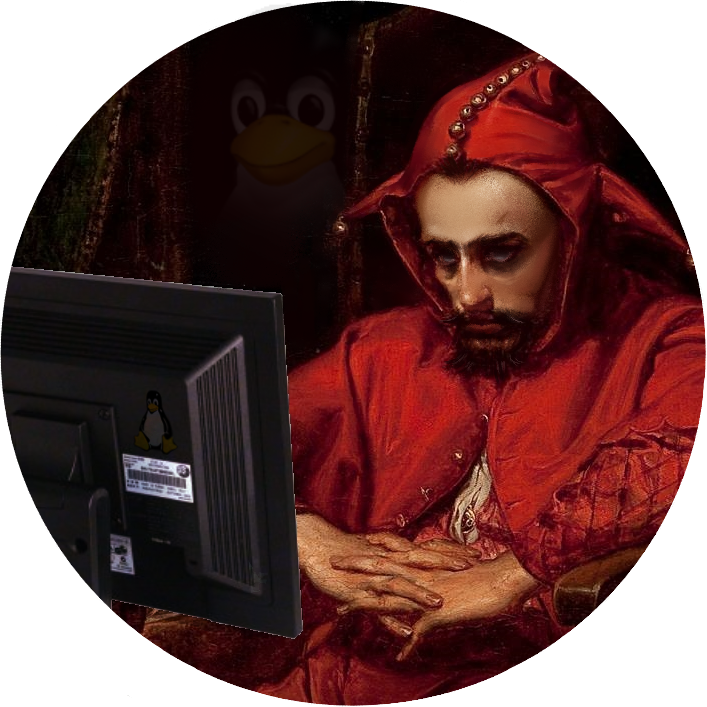Description

# Exercise #7

Complete the `dl.c` and `elf.c` programs.

### `dl.c`

• It should use `dlopen(3)` and `dlsym(3)` to open the `/lib64/libm.so.6` library and get the addresses for the `sin()` and `cos()` functions and compute and print the result of `sin(cos(M_PI))` where `M_PI` is the constant:

`#define M_PI 3.14159265358979323846 /* pi */`

Your completed program should not in any way be linked against the math library, but should be equivalent to:

``````#include <stdio.h>
#include <math.h>

int main(void)
{
printf("sin(cos(pi)) = %lf\n", sin(cos(M_PI)));
return 0;
}``````

### `elf.c`

• This program should print the header information for an ELF object ala: '`readelf -h <file>`'. To do this it should mmap the ELF file into the processes address space (i.e. the `mapfile()` function) and then the map the `Elf64_Ehdr` structure onto it and print out the information in the same format as the `readelf` program outputs.
Files